## 软件处理不了加腋怎么办？我来教你学手算!1、垂直加腋的构造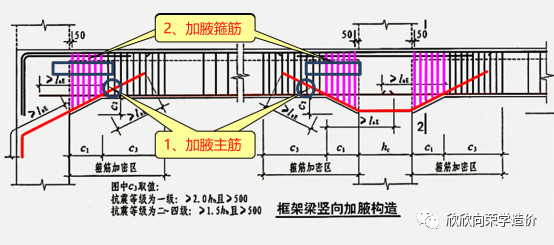2、案例图纸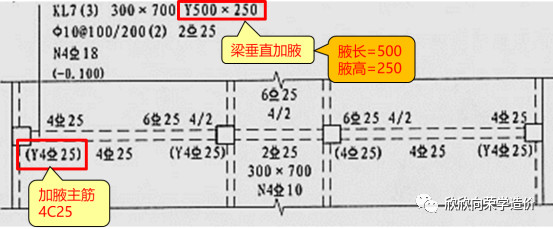从图纸可以得出：

（图集规定加腋箍筋同框架梁端部箍筋）

3、加腋主筋的计算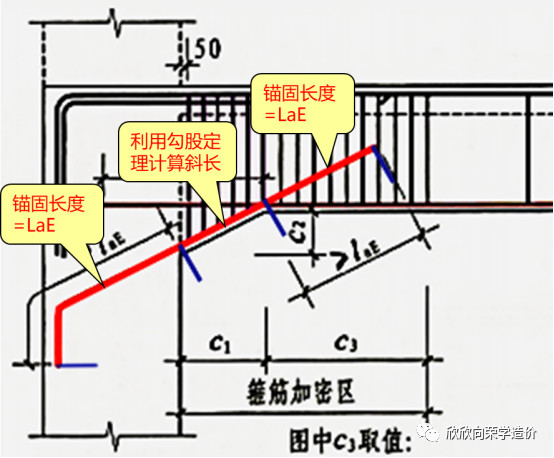把图纸的信息代入可以得到：

=锚固+斜长+锚固

=LaE+√500²+250²+LaE

=40*25+559+40*25

=2559mm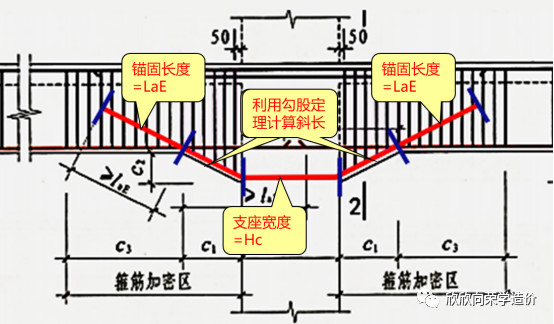4、箍筋的根数计算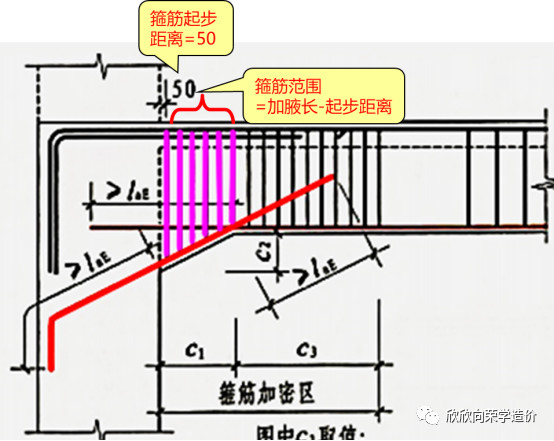=（腋长-起步）/加密间距+1

=（500-50）/100+1

=4.5向上取整+1

=6根

5、箍筋的长度计算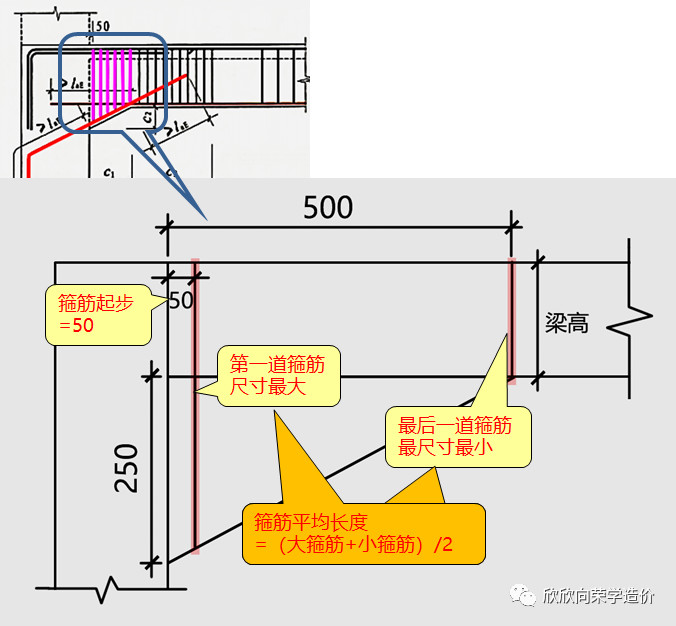（1）计算小箍筋

=（梁高+梁宽）*2-8*保护层+11.9d*2

=（700+300）*2-8*20+11.9*10*2

=2078mm

（2） 计算大箍筋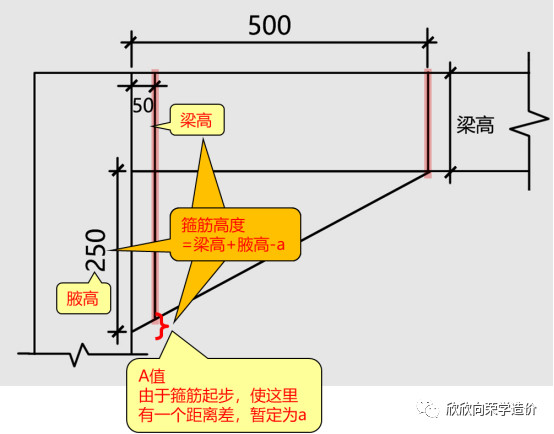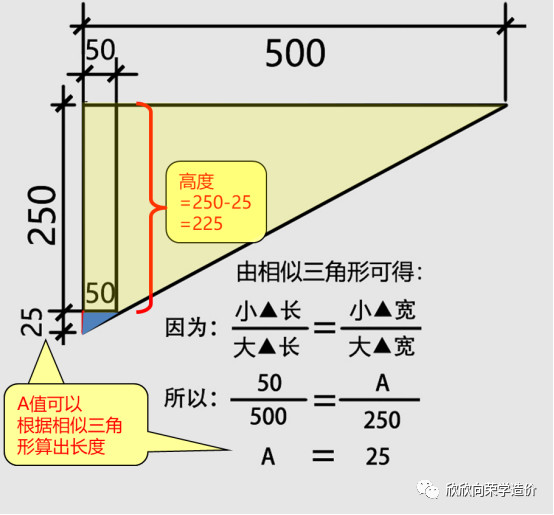=（梁高+225+梁宽）*2-8*保护层+11.9d*2

=（700+225+300）*2-8*20+11.9*10*2

=2528mm

（3）计算箍筋平均长度

=（大箍筋长度+小箍筋长度）/2

=（2528+2078）/2

=2303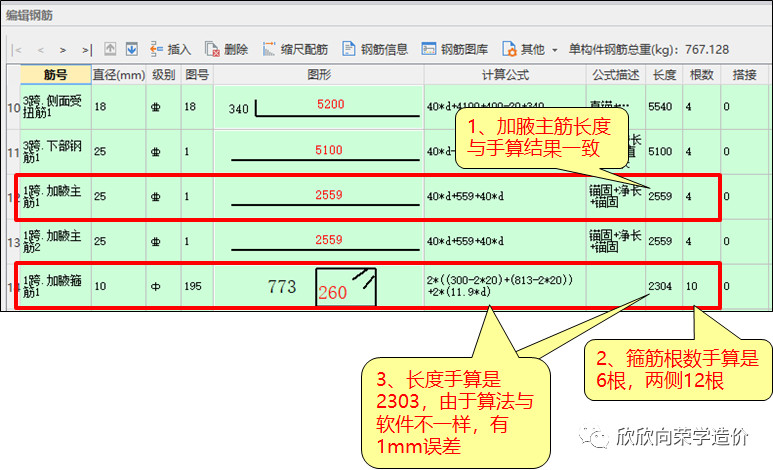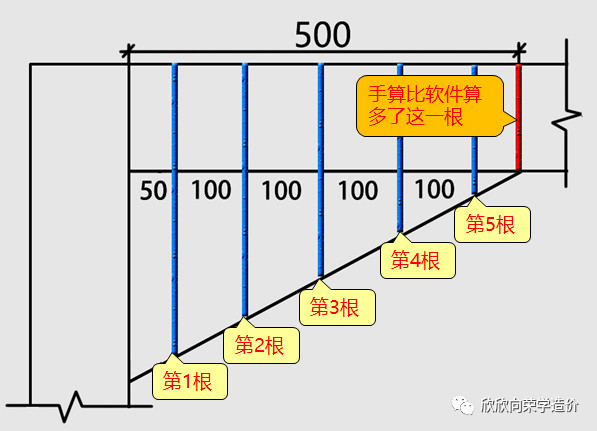1、水平加腋的构造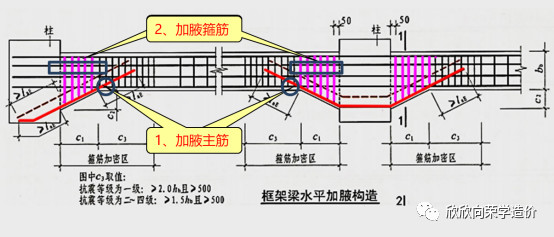2、案例图纸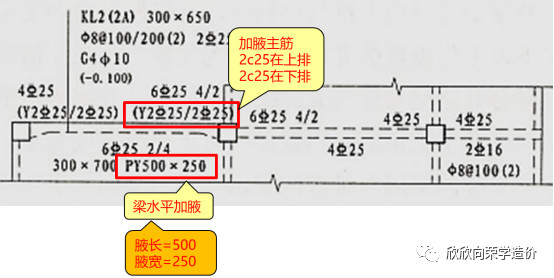（图集规定加腋箍筋同框架梁端部箍筋）

4、加腋主筋计算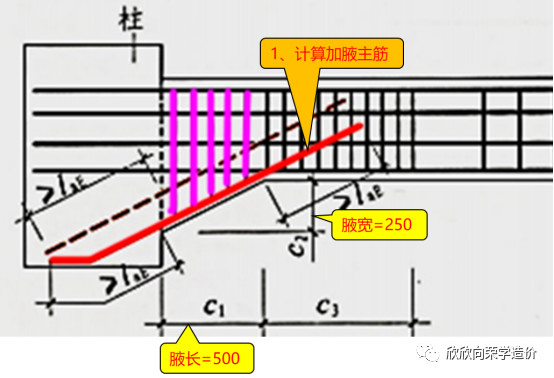=锚固+斜长+锚固

=LaE+√500²+250²+LaE

=40*25+559+40*25

=2559mm

2、箍筋计算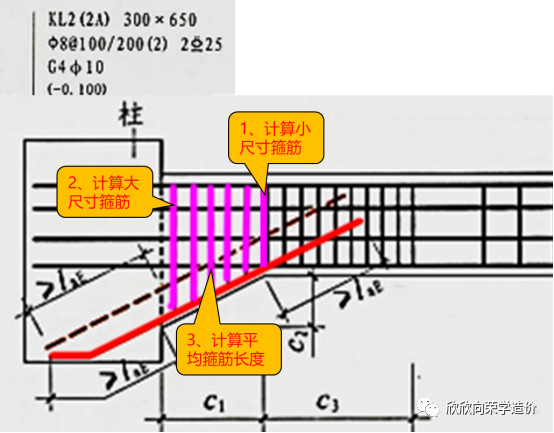=（梁高+梁宽）*2-8*保护层+11.9d*2

=（650+300）*2-8*20+11.9*10*2

=1978mm

=（梁高+225+梁宽）*2-8*保护层+11.9d*2

=（650+225+300）*2-8*20+11.9*10*2

=2428mm

=（大箍筋长度+小箍筋长度）/2

=（2428+1978）/2

=2203mm

=（腋长-起步）/加密间距+1

=（500-50）/100+1

=4.5向上取整+1

=6根

1、混凝土计算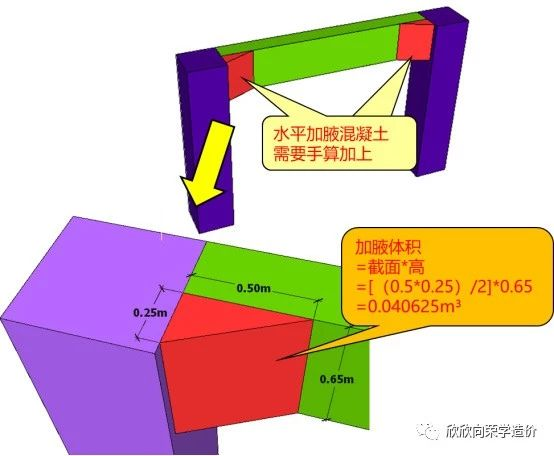0.040625*2=0.08125m³

2、模板计算

正常加腋梁的模板应该计算两侧的模板和底侧的模板，如图16所示。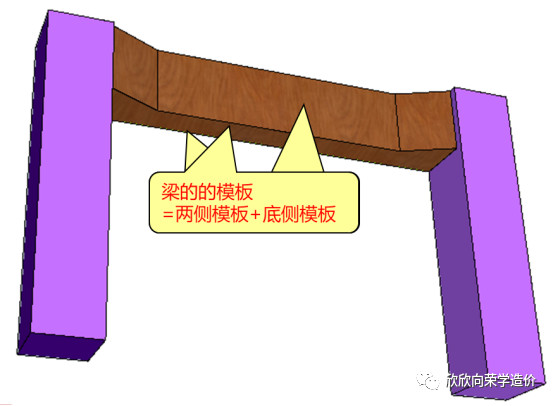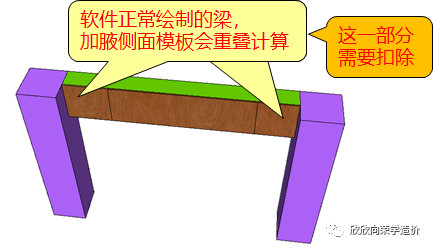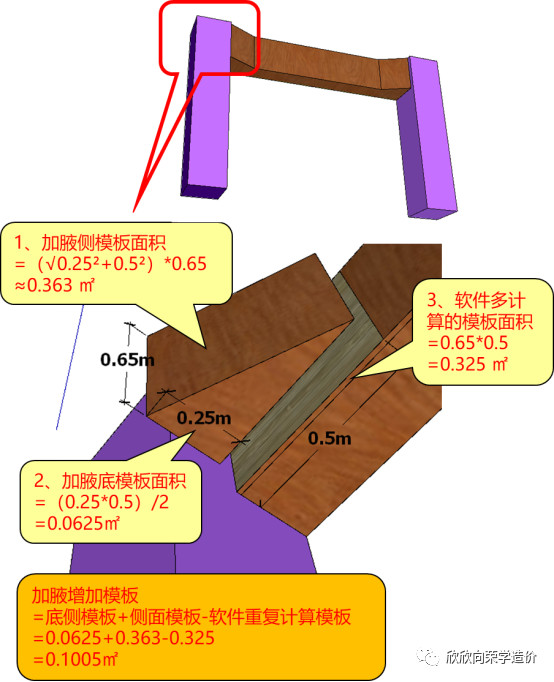处理水平加腋的最后一步就是把计算出来的工程量填在表格输入里，先把计算出来的加腋多出来的钢筋填上，如图18所示。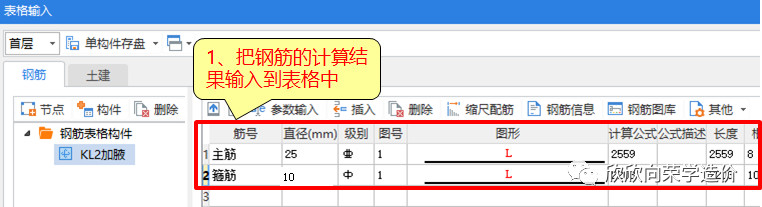然后把增加的混凝土和模板输入到表格输入里，如图20所示。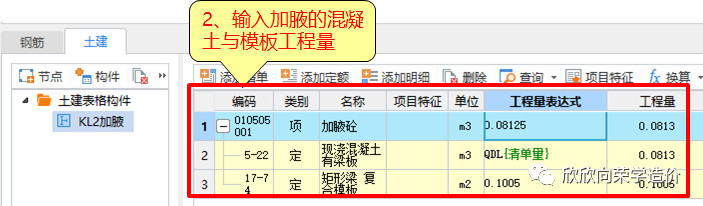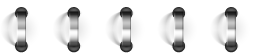2019.8.14

(作者) 内容检查中 未能发表

@

28条评论

@